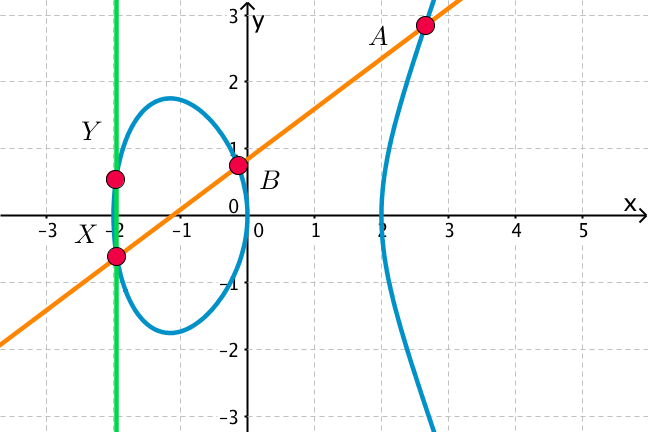## Want to keep learning?

This content is taken from the UNSW Sydney's online course, Maths for Humans: Linear, Quadratic & Inverse Relations. Join the course to learn more.
4.7

## UNSW SydneyMultiplication on a cubic curve

# Multiplication of points on conics and cubics (an advanced topic)

One of the reasons that mathematicians have been fascinated by cubic curves is that they have a remarkable algebraic structure which is not at all obvious. This is a novel way of “multiplying” points on a cubic curve through a curious geometrical construction.

In this (advanced) step, you will

• first learn about the simpler multiplication on a conic, which is also very interesting

• see how to multiply points on a cubic.

This is a bit of an advanced topic, but we think you may find it fun. Warning: the notion of multiplication that we are going to be seeing here is not at all the same as familiar multiplication! If you have GeoGebra on your computer, you can play around and actually follow the discussion with a specific example — a great way to get a feel for this remarkable phenomenon which is surprisingly connected to interesting number theory (including Fermat’s last theorem) and modern cryptography.

## Multiplying points on a parabola

Let’s begin with a simple but elegant way of “multiplying” points on a conic. we’ll start with the simplest conic, the parabola $$\normalsize{y=x^2}$$, and mark the simplest point, the origin $$\normalsize{O=[0,0]}$$ on it. Now take any two points on the conic, say $$\normalsize{A}$$ and $$\normalsize{B}$$. Draw the line through $$\normalsize{O}$$ parallel to $$\normalsize{AB}$$, and suppose that it meets the curve again at the point $$\normalsize{C}$$. Then we define

$\Large{A\times B=C}.$Q1 (M): If $$\normalsize{A=[1,1]}$$ and $$\normalsize{B=[2,4]}$$ what is the slope of the line $$\normalsize{AB}$$? What is the equation of the line through $$\normalsize{O}$$ parallel to $$\normalsize{AB}$$? What is the point $$\normalsize{C=A\times B}$$ in this case?

Q2 (C): Let’s be more general. Let $$\normalsize{A=[r,r^2]}$$ and $$\normalsize{B=[s,s^2]}$$ be two arbitrary points on the parabola. What is the slope of the line $$\normalsize{AB}$$? What is the equation of the line through $$\normalsize{O}$$ parallel to $$\normalsize{AB}$$? What is the point $$\normalsize{C=A\times B}$$ in this case?

## Multiplying points on a general conic

Now let’s go one step further: the same procedure for multiplying points works on a general conic, with $$\normalsize{O}$$ an arbitrary fixed point on it. We no longer require $$\normalsize{O}$$ to be the origin, but we do required it to be chosen once and for all. Then we define a (new) multiplication of points on the conic: to multiply $$\normalsize{A}$$ and $$\normalsize{B}$$, we draw the line through $$\normalsize{O}$$ parallel to $$\normalsize{AB}$$, and let it meet the conic also at the point $$\normalsize{C=A \times B}$$.What properties does this multiplication of points have? One of the properties is that $$\normalsize{O\times A=A}$$ for any point $$\normalsize{A}$$. Can you see why this is true? Another more subtle property is that the multiplication is associative, meaning that

$\Large{(A\times B)\times C=A\times(B\times C)}$

for any three points $$\normalsize{A}$$, $$\normalsize{B}$$, $$\normalsize{C}$$ on the conic. It is great fun to verify this yourself using GeoGebra. Make yourself a conic — GeoGebra can generate a unique conic through any five points — and then pick three points on that conic, and compute both sides of the above equation.

## Multiplying points on a cubic

Now we up the ante considerably by defining a multiplication of points on a particular cubic curve, say $$\normalsize{y^2=x^3-4x}$$. This definition is a bit more involved than in the conic situation, and the multiplication is considerably more mysterious!

Here is how to multiply two points $$\normalsize{A}$$ and $$\normalsize{B}$$ which lie on the curve. The line $$\normalsize{AB}$$ meets the curve in a third point, say $$\normalsize{X}$$. Now reflect $$\normalsize{X}$$ in the $$\normalsize{x}$$-axis to obtain $$\normalsize{Y}$$. Then define

$\Large{A\times B=Y}.$This does appear a curious operation, but it is still associative! That means that if we have three points $$\normalsize{A}$$, $$\normalsize{B}$$ and $$\normalsize{C}$$ on the curve, then

$\Large{(A\times B)\times C=A\times (B\times C)}.$

Q3 (C): If you multiply $$\normalsize{(A\times B)\times C}$$ in the figure below, which point do you get on the curve? What happens if you compute $$\normalsize{A\times (B\times C)}$$?You can get a good appreciation of the beauty of geometry by experimenting with different initial points, and verifying that the associative property always holds. Perhaps it appears that this is all just abstract geometry without any possible application — but in internet security, exactly this kind of multiplication plays a central role in what is called elliptic curve cryptography!

A1. The slope of the line is $$\normalsize m=\frac{4-1}{2-1}=3$$, so $$C$$ will lie on the line $$\normalsize y=3x$$. Solving $$\normalsize y=3x$$ and $$\normalsize y=x^2$$ we conclude that $$\normalsize x=3$$, and hence $$\normalsize C$$ is the point $$\normalsize [3,9]$$.
A2. The slope of the line is $$\normalsize m=\frac{s^2-r^2}{s-r}=s+r$$. If we now try to solve the equations $$\normalsize y=(s+r)x$$ and $$\normalsize y=x^2$$ we will find that the non-zero solution is $$\normalsize x=s+r$$. Hence we conclude that $$\normalsize C=A\times B$$ is the point $$\normalsize [s+r,(s+r)^2]$$. So the somewhat mysterious multiplication of points on the parabola corresponds exactly to just addition of $$\normalsize x$$-coordinates!
A3. Following the lines in the diagram we conclude that $$\normalsize A\times B=Y$$. If we repeat the process with $$\normalsize Y$$ and $$\normalsize C$$ we find $$\normalsize (A\times B)\times C= Y\times C = X$$.
If instead we were to start with $$\normalsize B$$ and $$\normalsize C$$ then we find $$\normalsize B\times C=Z$$. Applying this geometric construction to $$\normalsize A$$ and $$\normalsize Z$$ we find $$\normalsize A\times (B\times C)=A\times Z= X$$.